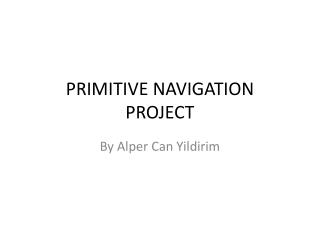DownloadDownload PresentationTélécharger la présentation- - - - - - - - - - - - - - - - - - - - - - - - - - - E N D - - - - - - - - - - - - - - - - - - - - - - - - - - -
##### Presentation Transcript

1. PRIMITIVE NAVIGATION PROJECT By Alper Can Yildirim

2. Aim : To find the latitude and longitude of Cambridge by tracking the shadows of a stick during different time periods of the same day.

3. Equipments • Foot long stick • CfA’s roof • A digital watch • Tape Measure

4. a straight wooden stick.  The shadow of the stick The surface

5. How do we calculate θ ? tan θ = length of the stick / length of the shadow So; θ = tan-1 ( Lstick / Lshadow)

6. Vertical Angle vs. Time of the Day Vertical Angle Time of the day (hours)

7. Taking the derivative y = -1.286x2 + 29.82x – 147.4 y’ = 2*(-1.286) x + 29.82 = 0 (setting it equal to zero to find the x and y coordinates of the vertex) x = LAN = 11.59 hours  11:35 AM Y= MH = 25.49°

8. Latitude from meridian height of sun Meridian height Latitude = 90o+Decl-Meridian height Due South

9. Latitude of Cambridge Latitude experiment = 90° - 21.87° - 25.49° = 42.64° Cambridge = 42.38° Error = 0.26° = 15.6’ Declination value for December 2 is taken from the website http://www.wsanford.com/~wsanford/exo/sundials/DEC_Sun.html Declination of sun @ December 2 = -21°52’ = -21.87°

10. Conversion to UTC • LAN = 11:35 • LAN + 5 hours + EoT = UTC • We need to find the EoT for December 2. from the equation of time graph.

11. Longitude of Cambridge • The difference between UTC and 12:00 will give us the longitude. • LAN + 5 hours + EoT = 11:35 + 5h + 10’= 16:45 • 16:45 – 12:00 = 4h 45’ = 4.75 h • 1 h = 15° • Therefore 4.75h = 71.25° • Cambridge = 71.11° • ERROR = 71.25°-71.11° = 0.14° = 8.4’